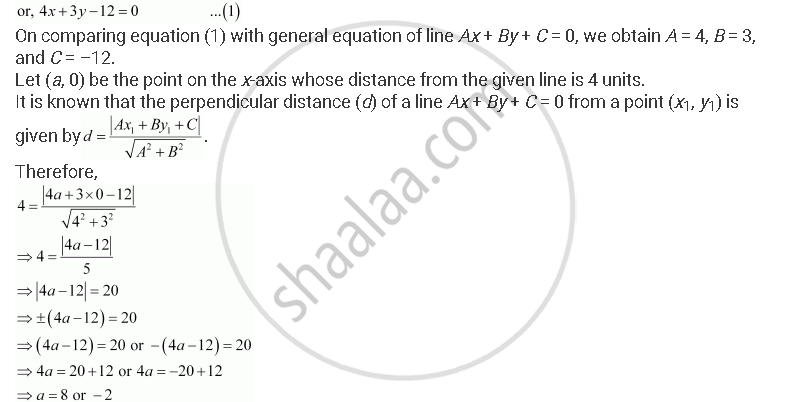CBSE (Arts) Class 11CBSE
Share

# Find the Points on The X-axis, Whose Distances from the X/3 +Y/4 = 1 Are 4 Units. - CBSE (Arts) Class 11 - Mathematics

ConceptDistance of a Point from a Line

#### Question

Find the points on the x-axis, whose distances from the x/3 +y/4 = 1  are 4 units.

#### Solution

The given equation of line is x/3 +y/4 = 1Thus, the required points on the x-axis are (–2, 0) and (8, 0).

Is there an error in this question or solution?

#### Video TutorialsVIEW ALL 

Solution Find the Points on The X-axis, Whose Distances from the X/3 +Y/4 = 1 Are 4 Units. Concept: Distance of a Point from a Line.
S